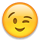# Physics problem

edited June 2013
Hi there, I am trying to use physics for the first time with orx.

So far I have drawn a basic closed room with walls, the ground and a sphere. I wanted the sphere to roll around the room as if it was a snooker ball.
But the result I got is that the ball start going one direction and back, non-stop, as if there a force was being applied in to one direction then another force was applied in the invese direction.

Here is the code section (I have tried apply force, apply torque and apply impulse, they all had the same result);:
``````orxVECTOR force;
force.fX = 0.0; //orx2F(-10.0);
force.fY = -10.0;
force.fZ = 0.0;
grid->beginPos.fZ = -0.1;
orxObject_SetPosition(sphere, &grid->beginPos);
orxObject_ApplyForce(sphere, &force, orxNULL);
orxObject_ApplyTorque(sphere, 50.0);
//orxObject_ApplyImpulse(sphere, &force, orxNULL);
``````

And the ini:
``````
[Basic_Sphere_Graphic]
Texture       = ../../art/sphere.png
Pivot = left top

[Basic_Sphere_FullPartList]
Type        = sphere
SelfFlags   = 0x0001; <= This defines our collision flags
CheckMask   = 0xFFFF; <= This defines the collision mask that will be testing on other objects
Solid       = true

[Basic_Sphere_Body]
PartList    = Basic_Sphere_FullPartList
Dynamic     = true
AllowSleep  = false

[Basic_Sphere_Object]
Graphic = Basic_Sphere_Graphic
Alpha   = 1.0
Body    = Basic_Sphere_Body

[Ground_Object]
Graphic         = Ground_Graphic
Alpha           = 1.0

[Ground_Graphic]
Texture       = ../../art/ground_colored.png
Pivot         = left top

[Wall_Body_FullPartList]
Type        = box
SelfFlags   = 0xFFFF; <= This defines our collision flags
CheckMask   = 0x0001; <= This defines the collision mask that will be testing on other objects
Solid       = true;

[Wall_Body]
PartList    = Wall_Body_FullPartList
Dynamic     = false
AllowSleep  = false

``````

Any insights?

• edited June 2013
Hi Knolan,

Sorry for the delay but I'm currently in the process of moving from San Francisco to Montreal and don't have much computer time if any.

Second thing: you shouldn't apply both a torque and a force, it's unnecessary and they might either work against each other or combine into undesired effects.
If you push your ball with a force, frictions will make it roll.
Good luck!My pleasure! Glad you got it working, as I said, physics-setting matters are always a bit tricky (and a lot of trials&errors)!